# Jump on the Number Line

January 7, 2011 —Comments Off

The real line runs from negative to positive infinity and consists of rational and irrational numbers. It generally appears horizontally, and every point corresponds to a real number. Also known as a number line in school, the real line is said to be one of the most useful ways to understand basic mathematics. Wolfram|Alpha can now aid you in learning the difference between x<-5 and x>5, or Abs[x]<2.

Wolfram|Alpha now graphs inequalities and points on the real line. This new feature in Wolfram|Alpha allows you to plot a single inequality or a list of multiple inequalities. Let’s start off simply and try “number line x<100”.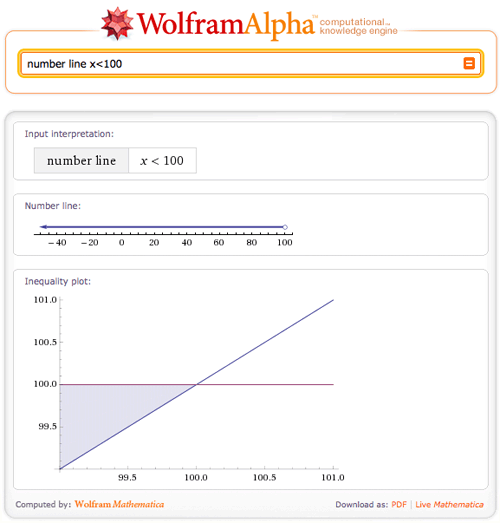You can easily see that this is the set of all real numbers from negative infinity to, but not including, 100.

What if you need to plot a more difficult inequality, like “number line 3x<7x^2+2”? This plot will show that the solutions to this inequality are all real numbers between negative and positive infinity.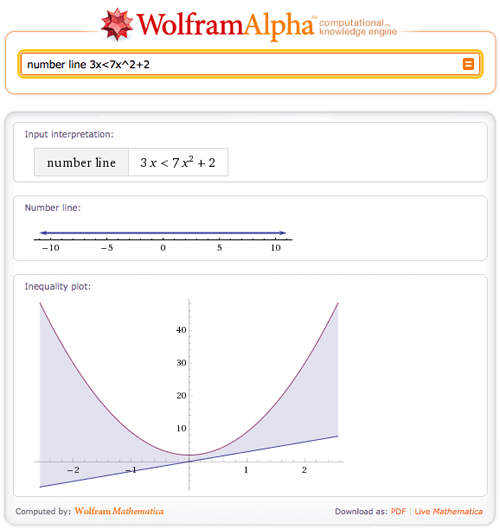Or try “-1<sin(x)<=1”: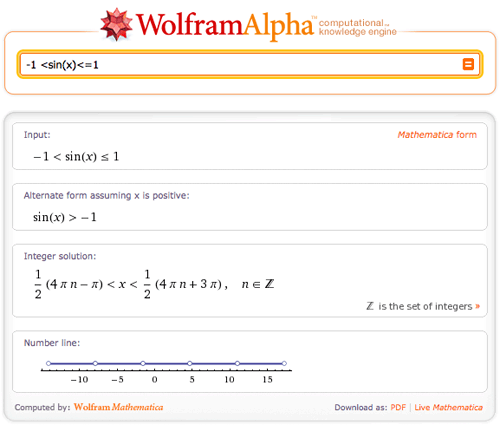Of course, Wolfram|Alpha can also plot a list of numbers on a number line. Try something like “e, Pi, 5, square root of 2”.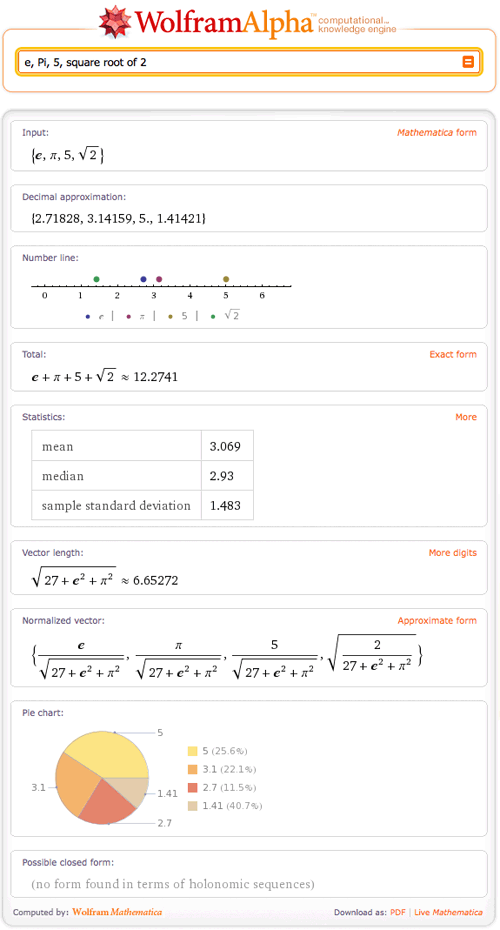This will nicely depict these values on the real line.

What if you want to plot more than one inequality? Just give Wolfram|Alpha a list, for example, “x<2, x>5x+7, x=10, x>=-2x”.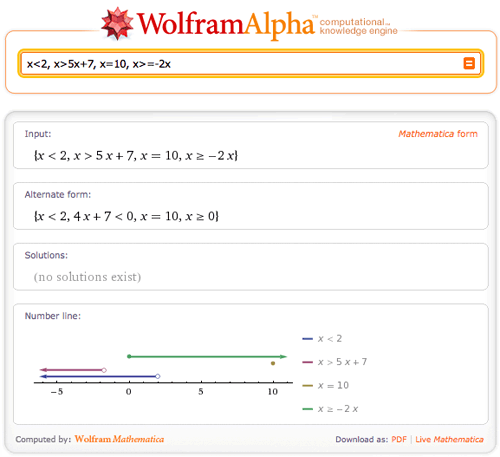Wolfram|Alpha now has the ability to plot 1D, 2D, and 3D graphics, so be sure to use it for all of your graphical needs!

Interesting idea, but wouldn’t it make sense to graph “steps” on the number line as arrows, so in other words “3-4” would give an arrow from 4 to 3?
And secondly shouldn’t the number line include imaginary values as well for the sake of completeness, although I don’t think it will serve that much of a purpose.

Posted by David Mulder January 10, 2011 at 10:27 am

Hello David,

Wolfram|Alpha doesn’t display imaginary numbers on the number line, as a traditional number line is designed to be a graphical representation of the real numbers. To see the imaginary numbers represented graphically, simply input the number you would like to see. Try, for example, the input “1 + i”, http://www.wolframalpha.com/input/?i=1%20%2B%20i&t=ff3tb02i . In regards to your second questions, we are looking into this. We’ve had several requests for this. I hope that we’ve answered your questions. Thank you!

Posted by The Wolfram|Alpha Team January 16, 2011 at 11:44 pm

HOW DOES ONE WRITE OUT THE INTERVAL NOTATION FOR THE ABOVE PROBLEM?

Posted by Sarah Leonard January 18, 2011 at 11:32 am